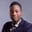Related Tags

python
community creator

# How to solve the "merging two packages" problemOsinachi Chukwujama

So, let’s say we have an algorithm problem with the following statement:

Given a package with a weight limit (limit) and an array (arr) of item weights, implement a function (getIndicesOfItemWeights) that finds two items whose sum of weights equals the weight limit (limit). Your function should return a pair [i, j] of the indices of the item weights, ordered such that i > j. If such a pair doesn’t exist, return an empty array.

## Problem analysis

Before writing any code in an interview or algorithmic problem, try to explain it in plain English or pseudocode.

### Parameters

1. limit
2. arr

### Requirements

1. Find two items in arr whose sum is limit
2. Return the indices of these items
3. The bigger index should be the first in the returned array.

The basic idea is to find a pair that adds up to the limit. The first solution you may think of is having a nested for-loop where you can try to find a pair that sums up to limit.

## Naive solution

def getIndicesOfItemWeights(arr, limit):
for num in arr:
numIndex = arr.index(num)
for index in range(numIndex+1, len(arr)):
if num + arr[index] == limit:
if num > arr[index]:
return [numIndex, index]
return [index, numIndex]
return []

print(getIndicesOfItemWeights([3, 4, 2, 6, 8], 12))

Di you notice how we used,

for index in range(numIndex+1, len(arr)):


in the second for-loop? We don’t want to compute the same pair twice, so we set the second loop to be an index ahead of the first. So, for the array [2, 4, 1, 5], we have the following pairs:

2, 4
2, 1
2, 5
4, 1
4, 5
1, 5


## Time complexity

The implementation above has a time complexity of $O(n^2)$ (quadratic) because of the for-loop. In the worst case, we will have to compute the last pair before returning an answer.

## More efficient solution

We aim to reduce the time-complexity from quadratic time to linear or logarithmic time. One way to approach this is to eliminate the nested loop. This means storing the results of the computation somewhere. A hashmap is be a good data structure for this. Since we are using Python, we will use a dictionary:

def getIndicesOfItemWeights(arr, limit):
hashmap = {}

for weight in arr:
hashmap[weight] = limit - weight

if hashmap[weight] in arr:
complementIndex = arr.index(hashmap[weight])
weightIndex = arr.index(weight)

if complementIndex > weightIndex:
return [complementIndex, weightIndex]
return [weightIndex, complementIndex]

return []

print(getIndicesOfItemWeights([3, 4, 2, 6, 8], 12))

The idea here is to transverse the array once and find the weight’s complement (limit - weight) at each iteration. We then check if that complement is in the array. If it is, we know we have succeeded. Remember, the ultimate goal is to find a weight-complement pair that adds up to limit. So, if the complement exists in the array, we can proceed to return:

1. The weight’s index
2. The complement’s index

This algorithm can be classified as a non-greedy algorithm – we can try to return a result as soon as possible.

## Time complexity

The time complexity of this algorithm is $O(n)$ because we transverse the array once.

## Space complexity

Hashmaps have an $O(n)$ space complexity because their size increases with the number of entries.

## Conclusion

You have learned a use case for hashmaps and how to move from a nested for-loop to a cleaner solution using a hashmap. The hashmap uses more space but offers faster implementation. Thanks for reading. Adios ✌🏾🧡.

RELATED TAGS

python
community creator

CONTRIBUTOROsinachi Chukwujama